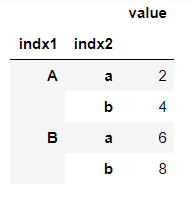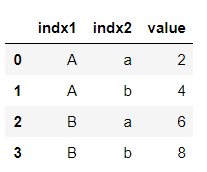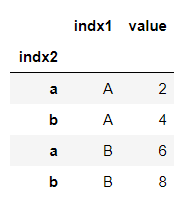Skip to content
Related Articles
Turn Pandas Multi-Index into column
• Last Updated : 24 Feb, 2021

Pandas DataFrame is a two-dimensional size-mutable, potentially heterogeneous tabular data structure with labeled axes (rows and columns). A multi-index dataframe has multi-level, or hierarchical indexing. We can easily convert the multi-level index into the column by the reset_index() method.

DataFrame.reset_index() is used to reset the index to default and make the index a column of the dataframe.

Step 1: Creating a multi-index dataframe.

Let’s see an example by making a multi-index dataframe first.

Code:

## Python3

 `import` `pandas as pd ` ` `  `# Creating index for muti-index dataframe ` `tuples ``=` `[(``'A'``, ``'a'``), (``'A'``, ``'b'``), (``'B'``, ``'a'``), (``'B'``, ``'b'``)] ` `index ``=` `pd.MultiIndex.from_tuples(tuples) ` ` `  `# Value corresponding to the index ` `data ``=` `[``2``, ``4``, ``6``, ``8``] ` ` `  `# Creating dataframe using 'data' and 'index' ` `df ``=` `pd.DataFrame(data ``=` `data, index ``=` `index, columns ``=` `[``'value'``]) ` `print``(df)`

Output:Step 2: Converting index into the column.

Here we can see the hierarchical indexing, we are going to convert it into a column using the reset_index() method.

## Python3

 `reset_df ``=` `df.reset_index() ` `print``(reset_df)`

Output:Here we can see that the index is default now and our multi-index now becomes columns with the default. We can also select which level of multi-index to reset using parameter level.

Code:

## Python3

 `# rest only index 'indx1' ` `reset_indx1 ``=` `df.reset_index(level``=``'indx1'``) ` `print``(reset_indx1)`

Output:Here, we can see that only indx1 is reset becomes a column, not both the indices. Also, notice that there is no default index in this case because there is still an index (indx2) left.

Attention geek! Strengthen your foundations with the Python Programming Foundation Course and learn the basics.

To begin with, your interview preparations Enhance your Data Structures concepts with the Python DS Course.

My Personal Notes arrow_drop_up
Recommended Articles
Page :